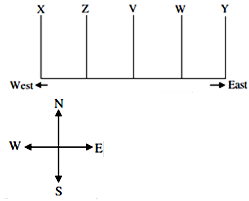# GATE Solved Paper 2017-19 - GATE 2017 Shift 2

>>>>>>>>GATE 2017 Shift 2

• A

1/5• B

7/30• C

1/4• D

4/15• Option : D
• Explanation :
Now we can solved by:
Ways to collect both Red = 3C2
Ways to collect both Green = 4C2
Ways to collect both Blue = 3C2
Total Ways = 10C2
P(Socks of Same Colour) = (3C2 + 4C2+ 3C2 ) / 10C2 = 4/15

• A

aversion, dislike• B• C

plunder, loot• D

yielding, resistant• Option : D
• Explanation :
The following are synonyms to each other:
1) aversion, dislike
3) plunder, loot
Or
Yield means to give in and resistance means to not give in.
Rest are all synonyms.
Hence, (D) is correct.

• A

V• B

W• C

X• D

Y• Option : A
• Explanation :
From the given data, the following is formed∴ The building ‘V’ is in the middle

• A

12• B

15• C

18• D

19• Option : B
• Explanation :
Total marks in the test = 100
For multiple choice questions = 3 marks
For essay questions = 11 marks
Option (A)
Marks for multiple choice questions = 12 × 3 = 36
Marks for essay type questions = 100 – 36 = 64
64 is not divisible by 11
∴ Option (A) is not correct.
Option (B)
Marks for multiple choice questions = 15 × 3 = 45
Marks for essay type questions = 100 – 45 = 55/11 = 11
Essay type questions are 5 No’s
∴ Option (B) is correct
Option (C)
Marks for multiple choice questions = 18 × 3 = 54
Marks for essay type questions = 100 – 54 = 46
46 is not divisible by 11
∴ Option (C) is not correct.
Option (D)
Marks for multiple choice questions = 19 × 3 = 57
Marks for essay type questions = 100 – 57 = 43
46 is not divisible by 11
∴ Option (D) is not correct.

• A

enough bright• B

bright enough• C

as enough bright• D

bright as enough• Option : B
• Explanation :
Saturn is bright enough to be seen on a clear night with the naked eye
Related Quiz.
GATE 2017 Shift 2